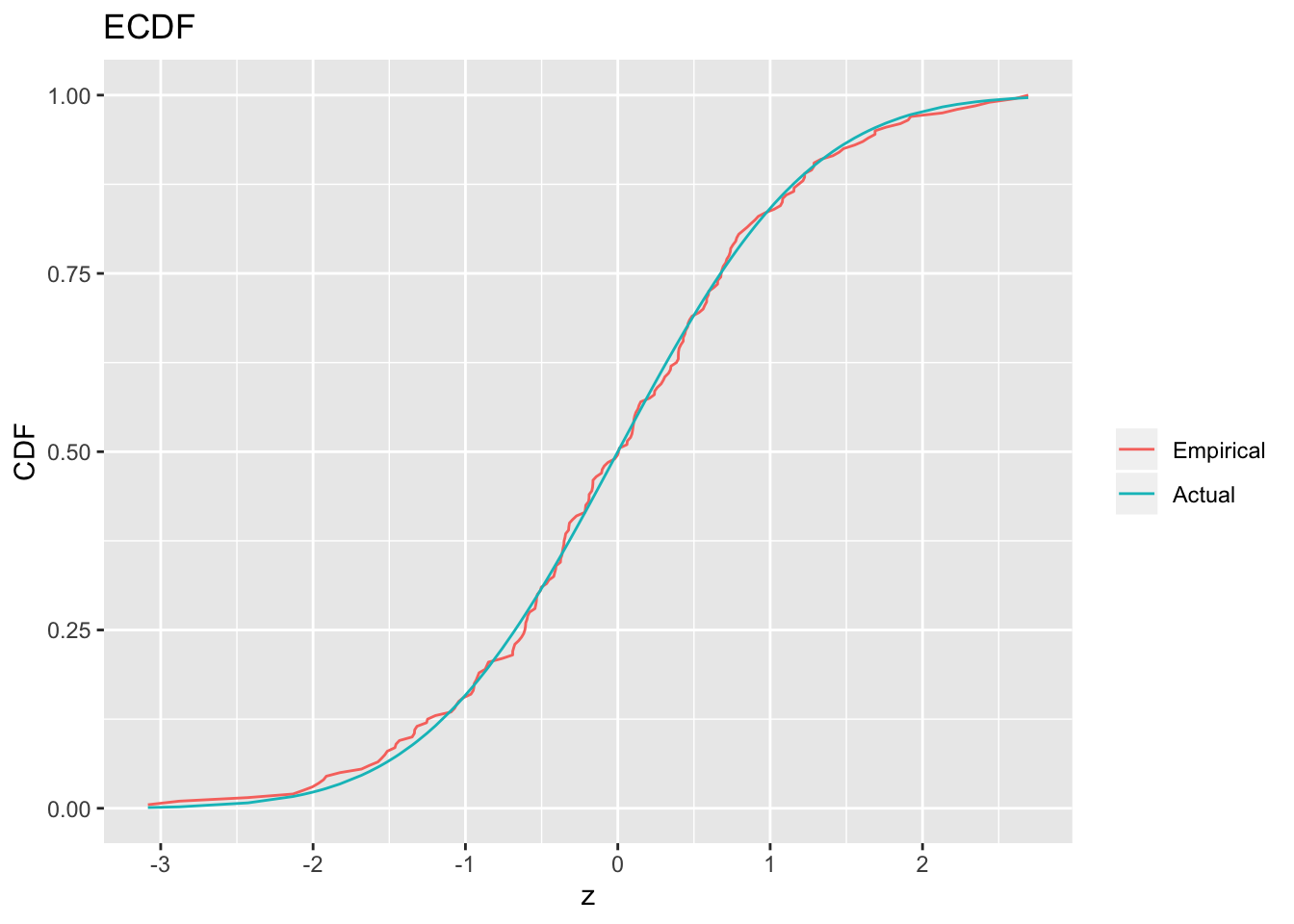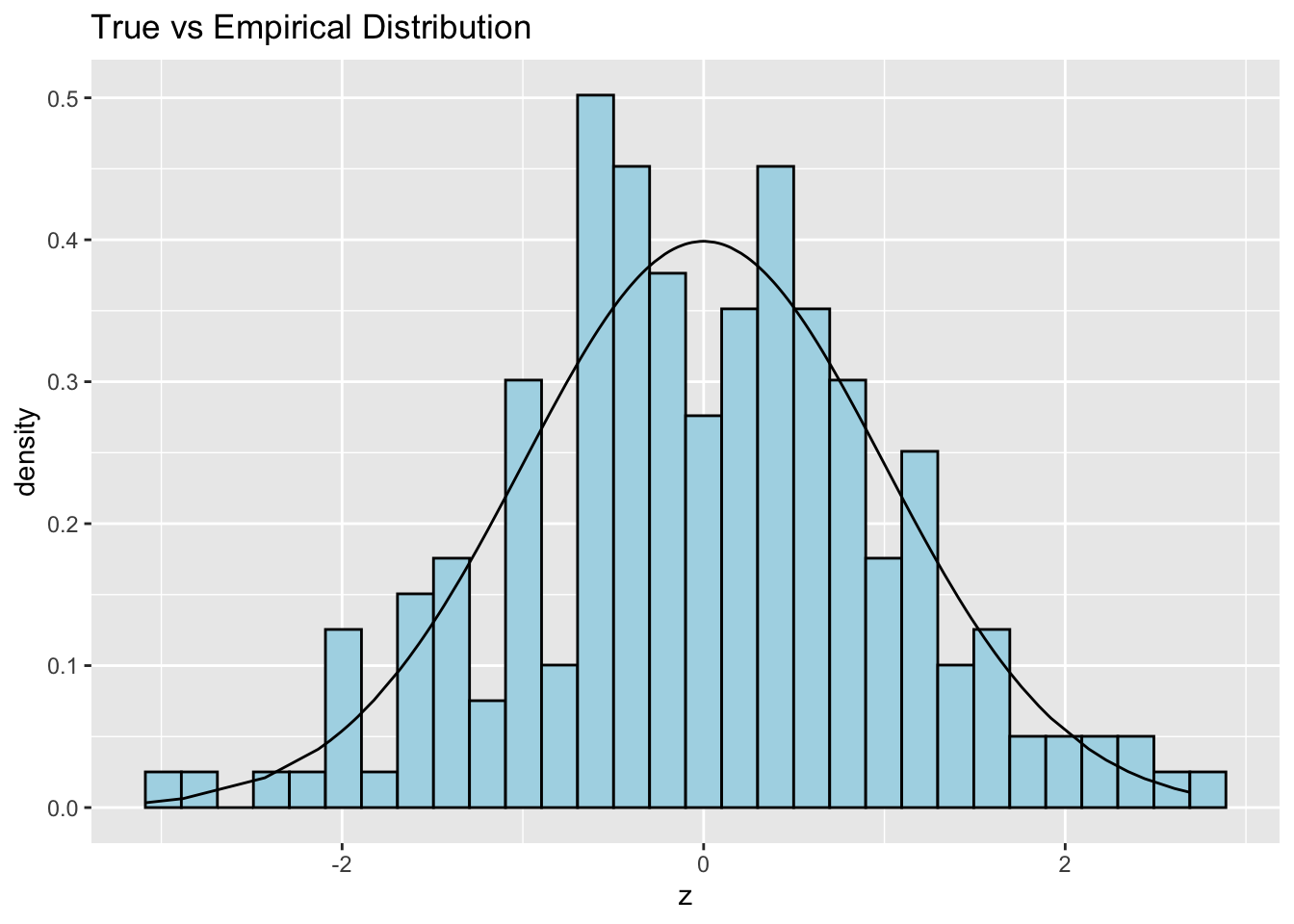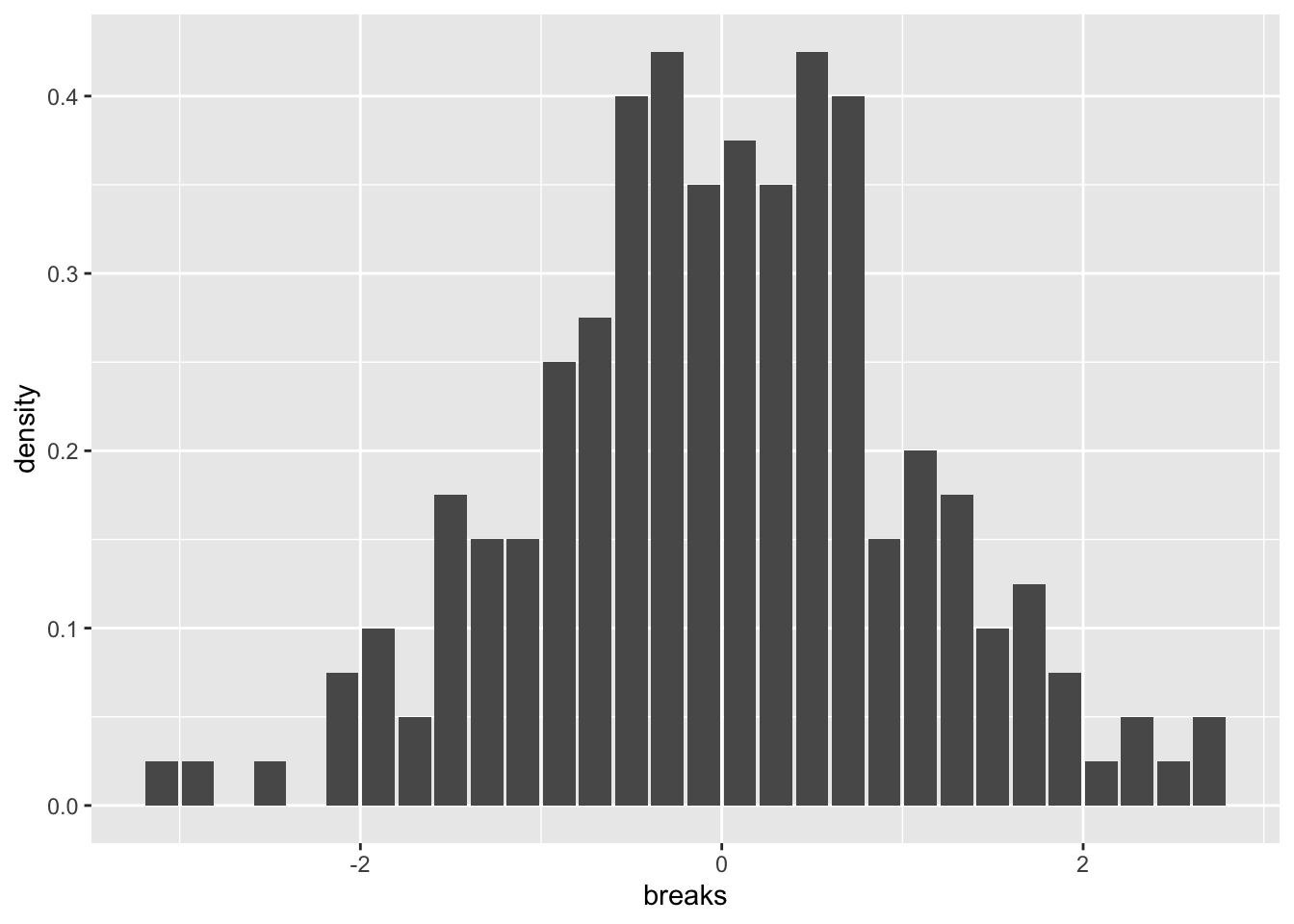# Last week: Tidyverse II: tidyr

• tidyr is a package for manipulating the structure of data frames
• gather(): make wide data longer
• spread(): make long data wider
• unite() and separate(): combine or split columns
• dplyr has advanced functionality that mirrors SQL
• group_by(): create groups of rows according to a condition
• summarize(): apply computations across groups of rows
• *_join() where * = inner, left, right, or full: join two data frames together according to common values in certain columns, and * indicates how many rows to keep

# Part I

Simulation basics

# Why simulate?

R gives us unique access to great simulation tools (unique compared to other languages). Why simulate? Welcome to the 21st century! Two reasons:

• Often, simulations can be easier than hand calculations
• Often, simulations can be made more realistic than hand calculations

# Sampling from a given vector

To sample from a given vector, use sample()

sample(x = letters, size = 10) # Without replacement, the default
##   "f" "t" "q" "p" "a" "z" "g" "j" "w" "n"
sample(x = c(0,1), size = 10, replace = TRUE) # With replacement
##   0 1 0 0 1 0 0 0 1 0
sample(x = 10) # Arguments set as x=1:10, size=10, replace=FALSE
##    5  8  4  7  2  3  1  6  9 10

# Random number generation

To sample from a normal distribution, we have the utility functions:

• rnorm(): generate normal random variables
• pnorm(): normal distribution function, $$\Phi(x)=P(Z \leq x)$$
• dnorm(): normal density function, $$\phi(x)= \Phi'(x)$$
• qnorm(): normal quantile function, $$q(y)=\Phi^{-1}(y)$$, i.e., $$\Phi(q(y))=y$$

Replace “norm” with the name of another distribution, all the same functions apply. E.g., “t”, “exp”, “gamma”, “chisq”, “binom”, “pois”, etc.

# Random number examples

Standard normal random variables (mean 0 and variance 1)

n <- 200
z <- rnorm(n, mean = 0, sd = 1) # These are the defaults for mean, sd
mean(z)  # Check: sample mean is approximately 0
##  0.006089724
var(z)   # Check: sample variance is approximately 1
##  1.092156

# Estimated distribution function

To compute empirical cumulative distribution function (ECDF)—the standard estimator of the cumulative distribution function—use ecdf()

library(tidyverse)
ecdf_fun <- ecdf(z) # Create the ECDF
class(ecdf_fun) # It's a function!
##  "ecdf"     "stepfun"  "function"
ecdf_fun(0)
##  0.495
# We can plot it

gaussian_data <- data.frame(z = sort(z)) %>%
mutate(ecdf = ecdf_fun(z),
true = pnorm(z))

gaussian_data_lines <- gaussian_data %>%
gather("line", "value",
ecdf, true, -z) %>%
mutate(line = forcats::fct_recode(line,
Empirical = "ecdf",
Actual = "true"))

ggplot(gaussian_data_lines, aes(x = z, y = value, color = line)) +
geom_line() +
labs(y = "CDF",
title = "ECDF",
color = "")# Estimated density function

To compute histogram —a basic estimator of the density based on binning—use hist()

hist_obj <- hist(z, breaks = 30, plot = FALSE) # base R function
class(hist_obj) # It's a list
##  "histogram"
hist_obj$breaks # These are the break points that were used ##  -3.2 -3.0 -2.8 -2.6 -2.4 -2.2 -2.0 -1.8 -1.6 -1.4 -1.2 -1.0 -0.8 -0.6 ##  -0.4 -0.2 0.0 0.2 0.4 0.6 0.8 1.0 1.2 1.4 1.6 1.8 2.0 2.2 ##  2.4 2.6 2.8 hist_obj$density # These are the estimated probabilities
##   0.025 0.025 0.000 0.025 0.000 0.075 0.100 0.050 0.175 0.150 0.150
##  0.250 0.275 0.400 0.425 0.350 0.375 0.350 0.425 0.400 0.150 0.200
##  0.175 0.100 0.125 0.075 0.025 0.050 0.025 0.050
# We can plot it
gaussian_data <- gaussian_data %>%
mutate(PDF = dnorm(z))

ggplot(gaussian_data, aes(x = z, y = ..density..)) +
geom_histogram(bins = 30,
fill = "lightblue",
col = "black") +
geom_line(aes(y = PDF)) +
labs(title = "True vs Empirical Distribution")hist_data <- data.frame(breaks = hist_obj$breaks[1:(length(hist_obj$breaks) - 1)] + .1,
density = hist_obj\$density)

ggplot(hist_data, aes(x = breaks, y = density)) +
geom_histogram(stat = "identity")
## Warning: Ignoring unknown parameters: binwidth, bins, pad# Tangent on estimation (did you know?)

Interesting statistical facts:

• When you have lots of data, the empirical distribution function will always be close to the actual distribution function (Glivenko-Cantelli, “Fundamental Theorem of Statistics”)
• When you have lots of data, the histogram estimator is not always close to the density function, only when the underlying density is quite smooth
• In general, density estimation is tricky, there are many estimators beyond histograms worth studying/knowing about; e.g., density() uses a kernel density estimator (gives a function) and you’ve already seen geom_density

# Part II

Pseudorandomness and seeds

# Same function call, different results

Not surprisingly, we get different draws each time we call rnorm()

mean(rnorm(n))
##  -0.06128671
mean(rnorm(n))
##  0.06798459
mean(rnorm(n))
##  0.0256657
mean(rnorm(n))
##  -0.07122027

# Is it really random?

Random numbers generated in R (in any language) are not “truly” random; they are what we call pseudorandom

• These are numbers generated by computer algorithms that very closely mimick “truly” random numbers
• The study of such algorithms is an interesting research area in its own right!
• The default algorithm in R (and in nearly all software languages) is called the “Mersenne Twister”
• Type ?Random in your R console to read more about this (and to read how to change the algorithm used for pseudorandom number generation, which you should never really have to do, by the way)

# Setting the random seed

All pseudorandom number generators depend on what is called a seed value

• This puts the random number generator in a well-defined “state”, so that the numbers it generates, from then on, will be reproducible
• The seed is just an integer, and can be set with set.seed()
• The reason we set it: so that when someone else runs our simulation code, they can see the same—albeit, still random—results that we do

# Seed examples

# Getting the same 5 random normals over and over
set.seed(0); rnorm(5)
##   1.2629543 -0.3262334  1.3297993  1.2724293  0.4146414
set.seed(0); rnorm(5)
##   1.2629543 -0.3262334  1.3297993  1.2724293  0.4146414
set.seed(0); rnorm(5)
##   1.2629543 -0.3262334  1.3297993  1.2724293  0.4146414

# Different seeds, different numbers
set.seed(1); rnorm(5)
##  -0.6264538  0.1836433 -0.8356286  1.5952808  0.3295078
set.seed(2); rnorm(5)
##  -0.89691455  0.18484918  1.58784533 -1.13037567 -0.08025176
set.seed(3); rnorm(5)
##  -0.9619334 -0.2925257  0.2587882 -1.1521319  0.1957828

# Each time the seed is set, the same sequence follows (indefinitely)
set.seed(0); rnorm(3); rnorm(2); rnorm(1)
##   1.2629543 -0.3262334  1.3297993
##  1.2724293 0.4146414
##  -1.53995
set.seed(0); rnorm(3); rnorm(2); rnorm(1)
##   1.2629543 -0.3262334  1.3297993
##  1.2724293 0.4146414
##  -1.53995
set.seed(0); rnorm(3); rnorm(2); rnorm(1)
##   1.2629543 -0.3262334  1.3297993
##  1.2724293 0.4146414
##  -1.53995

# Part III

Iteration and simulation

# Drug effect model

• Let’s start with a motivating example: suppose we had a model for the way a drug affected certain patients
• All patients will undergo chemotherapy. We believe those who aren’t given the drug experience a reduction in tumor size of percentage $X_{\mathrm{no\,drug}} \sim 100 \cdot \mathrm{Exp}(\mathrm{mean}=R), \;\;\; R \sim \mathrm{Unif}(0,1)$
• And those who were given the drug experience a reduction in tumor size of percentage $X_{\mathrm{drug}} \sim 100 \cdot \mathrm{Exp}(\mathrm{mean}=2)$ (Here $$\mathrm{Exp}$$ denotes the exponential distribution, and $$\mathrm{Unif}$$ the uniform distribution)

# What would you do?

What would you do if you had such a model, and your scientist collaborators asked you: how many patients would we need to have in each group (drug, no drug), in order to reliably see that the average reduction in tumor size is large?

• Answer used to be: get out your pen and paper, make some approximations
• Answer is now: simulate from the model, no approximations required!

# So, let’s simulate!

# Simulate, supposing 60 subjects in each group
set.seed(0)
n <- 60
mu_drug <- 2
mu_nodrug <- runif(n, min = 0, max = 1)
x_drug <- 100 * rexp(n, rate = 1/mu_drug)
x_nodrug <- 100 * rexp(n, rate = 1/mu_nodrug)

sim_data <- data.frame(x = c(x_drug, x_nodrug),
class = factor(rep(c("Drug","No Drug"), each = 60)))

sim_data %>%
ggplot(aes(x = x, y = ..density.., color = class)) +
geom_histogram(aes(fill = class),
alpha = .5, color = "black",
position = "identity") +
geom_density(aes(color = class))
## stat_bin() using bins = 30. Pick better value with binwidth.